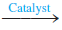# Problem: Ethene is converted to ethane by the reactionC2H4(g) + H2(g)     C2H6(g)C2H4 flows into a catalytic reactor at 25.0 atm and 300.°C with a flow rate of 1000. L/min. Hydrogen at 25.0 atm and 300.°C flows into the reactor at a flow rate of 1500. L/min. If 15.0 kg C2H6 is collected per minute, what is the percent yield of the reaction?

###### FREE Expert Solution

This problem requires that we use the ideal gas law first and then do stoichiometric calculations.

We will solve this problem through these steps:

• Use the ideal gas law to find the moles of ethene and hydrogen.
• Find the limiting reactant and theoretical yield.
• Find percent yield using the actual and theoretical yield.

79% (237 ratings)###### Problem Details

Ethene is converted to ethane by the reaction

C2H4(g) + H2(g)C2H6(g)

C2H4 flows into a catalytic reactor at 25.0 atm and 300.°C with a flow rate of 1000. L/min. Hydrogen at 25.0 atm and 300.°C flows into the reactor at a flow rate of 1500. L/min. If 15.0 kg C2H6 is collected per minute, what is the percent yield of the reaction?